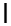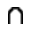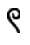11,052 Pages

This page lists the various numeral systems used in ancient times.

## Egyptian numerals

The Egyptian numerals were invented in ancient Egypt about 3000 BC.

 Value 1 10 100 1,000 10,000 100,000 1,000,000 HieroglyphEgyptian numerals system uses additive principle, for example:

The glyphs for addition and subtraction areand, respectively.

## Greek numerals

### Attic numerals

The Attic numerals appeared in the 7th century BC.

 Value Symbol Greek numeral 1 Ι μονὰς(monas) 5 Π πέντε(pente) 10 Δ δέκα (deka) 100 Η ἑκατόν (hɛkaton) 1,000 Χ χιλιάς (kilias) 10,000 Μ μύριον (myrion)

### Ionic numerals

In the 4th century BC the attic numerals were replaced by the ionic numerals.

 1 αʹ 10 ιʹ 100 ρʹ 1,000 ͵α 2 βʹ 20 κʹ 200 σʹ 2,000 ͵β 3 γʹ 30 λʹ 300 τʹ 3,000 ͵γ 4 δʹ 40 μʹ 400 υʹ 4,000 ͵δ 5 εʹ 50 νʹ 500 φʹ 5,000 ͵ε 6 ϛʹ 60 ξʹ 600 χʹ 6,000 ͵ϛ 7 ζʹ 70 οʹ 700 ψʹ 7,000 ͵ζ 8 ηʹ 80 πʹ 800 ωʹ 8,000 ͵η 9 θʹ 90 ϟʹ 900 ϡʹ 9,000 ͵θ

Greek numerals system uses additive principle, for example: ͵θτπεʹ = 9000+300+80+5 = 9,385

Ten thousand (one myriad) ancient greeks wrote as "M" and quantity of myriads they wrote before "M". For example:

͵θτπεM͵θτπεʹ = 93,859,385

Thus, the largest number which ancient greeks could write was ,θϡϟθM,θϡϟθ' = 99,999,999.

## Notation of Archimedes

Earliest googological notation in human history was invented by Archimedes (c. 287 – c. 212 BC) with aim to calculate how much grains of sand the Universe can contain (in supposition, that all universe filled by sand).

### Definition

All natural numbers up to myriada of myriads (100,000,000) are first numbers (ἀριθμοὶ ἐς τὰς μυρίας μυριάδας πρώτοι καλουμένοι). The unit of (n+1)ths numbers is myriada of myriads of n-ths numbers. And so on up to 100,000,000-ths numbers.

### Examples

• ιʹ μονάδες τῶν δευτέρων ἀριθμῶν = ten of units of second numbers $$=10\times 10^{8\times(2-1)}= 10^{9}$$
• μυρίαι μυριάδες τῶν δευτέρων ἀριθμῶν = myriad of myriads of second numbers=αʹ μονάδα τῶν τρίτων ἀριθμῶν = one unit of third order $$=10^{8\times(3-1)}= 10^{16}$$
• ιʹ μυριάδες τῶν τρίτων ἀριθμῶν = ten myriads of third numbers $$=10\times10^4\times10^{8\times(3-1)}= 10^{21}$$
• ιʹ μονάδες τῶν πέμπτων ἀριθμῶν = ten of units of fifth numbers $$=10\times 10^{8\times(5-1)}= 10^{33}$$
• ιʹ μυριάδες τῶν ἕκτων ἀριθμῶν = ten myriads of sixth numbers $$=10\times10^4\times10^{8\times(6-1)}=10^{45}$$
• ͵α μονάδες τῶν ἑβδόμων ἀριθμῶν = thousand of units of seventh numbers $$=10^3\times10^{8\times(7-1)}=10^{51}$$ = χιλίαι μονάδες τῶν ἑβδόμων ἀριθμῶν
• ͵α μυριάδες τῶν ὀγδόων ἀριθμῶν = thousand of myriads of eighth numbers= $$=10^3\times10^4\times10^{8\times(8-1)}=10^{63}$$
• ͵θτπεʹ μονάδες τῶν μυριακισμυριοστῶν ἀριθμῶν = 9,385 units of 100,000,000-ths numbers = $$9,385 \times 10^{799,999,992}$$

In general, each natural number up to $$10^{800,000,000}$$ can be uniquely written in this form:

$$N=\sum_{i=1}^k$$(Number of myriads of i-ths numbers+Number of units of i-ths numbers)

### Extension

All natural numbers up to myriada of myriads of 100,000,000ths numbers are numbers of first period (ἀριθμοὶ πρώτας περιόδου). The unit of first numbers of (n+1)th period is myriada of myriads of 100,000,000ths numbers of the n-th period.

μυρίαι μυριάδες τᾶς δευτέρας περιόδου πρώτων ἀριθμῶν μονὰς καλείσθω τᾶς δευτέρας περιόδου δευτέρων ἀριθμῶν

= the myriad of myriads of first numbers of 2nd period is equal to the unit of 2-ths numbers of 2nd period $$=10^{8\times 10^8 +8}$$

$$10^{16\times 10^8}$$ is unit of first numbers of 3rd period

$$10^{24\times 10^8}$$ is unit of first numbers of 4th period

$$10^{32\times 10^8}$$ is unit of first numbers of 5th period

and so on, up to:

μυριακισμυριοστᾶς περιόδου μυριακισμυριοστῶν ἀριθμῶν μυρίας μυριάδας

= myriad of myriads of 100,000,000th numbers of 100,000,000th period $$=10^{8\times 10^{16}}$$

## Roman numerals

 Symbol I V X L C D M Value 1 5 10 50 100 500 1,000

Roman numerals system uses additive principle, for example:

MMMCCCXXXIII=MMM+CCC+XXX+III=3000+300+30+3=3,333.

Subtractive notation is also used, in the following cases:

I placed before V or X reduces number by one, X placed before L or C reduces number by ten, C placed before D or M reduces number by hundred,

The largest number representible in Roman numerals is:

MMMMCMXCIX = 4,000+(1,000-100)+(100-10)+(10-1) = 4,000+900+90+9 = 4,999.

## Chinese numerals

Chinese numerals are numerals of ancient and modern usage in China. The invention of chinese numerals and math calculations traditionally ascribed to the mythological Yellow Emperor (2698–2598 BC).

### The numbers of everyday usage

 Chinese Characters (Simplified) Value Pinyin (Standard Chinese/Mandarin) 〇 0 líng 一 1 yī 二 2 èr 三 3 sān 四 4 sì 五 5 wǔ 六 6 liù 七 7 qī 八 8 bā 九 9 jiǔ 十 10 shí 百 100 bǎi 千 1,000 qiān 万 10,000 wàn

### Multiplicative principle

Chinese numerals system uses a multiplicative principle, for example:

• 一千一百五十八 = 1,158
• 八 = 8
• 八十八 = 88
• 八百八十八 = 888
• 八千八百八十八 = 8,888
• 八千〇八十 = 8,080

### Notations for large numbers

Notation 1:

• $$a_0=10^4$$
• $$a_{n+1}=a_n\cdot10$$

Notation 2:

• $$a_0=10^4$$
• $$a_{n+1}=a_n\cdot10^4$$

Notation 3:

• $$a_0=10^8$$
• $$a_{n+1}=a_n\cdot10^8$$

Notation 4:

• $$a_0=10^4$$
• $$a_{n+1}=a_n^2$$

### Names and values of the large numbers

 Chinese Characters (Simplified) Pinyin (Standard Chinese/Mandarin) N1 N2 N3 N4 万 wàn $$10^4$$ $$10^4$$ $$10^4$$ $$10^4$$ 亿 yì $$10^5$$ $$10^{8}$$ $$10^{8}$$ $$10^{8}$$ 万亿 zhào $$10^6$$ $$10^{12}$$ $$10^{16}$$ $$10^{16}$$ 京 jīng $$10^7$$ $$10^{16}$$ $$10^{24}$$ $$10^{32}$$ 垓 gāi $$10^8$$ $$10^{20}$$ $$10^{32}$$ $$10^{64}$$ 秭 zǐ $$10^9$$ $$10^{24}$$ $$10^{40}$$ $$10^{128}$$ 穰 ráng $$10^{10}$$ $$10^{28}$$ $$10^{48}$$ $$10^{256}$$ 沟 gōu $$10^{11}$$ $$10^{32}$$ $$10^{56}$$ $$10^{512}$$ 涧 jiàn $$10^{12}$$ $$10^{36}$$ $$10^{64}$$ $$10^{1,024}$$ 正 zhèng $$10^{13}$$ $$10^{40}$$ $$10^{72}$$ $$10^{2,048}$$ 载 zǎi $$10^{14}$$ $$10^{44}$$ $$10^{80}$$ $$10^{4,096}$$

## Avatamsaka Sutra's notation

In Avatamsaka Sutra, written in the India before 5 century AD, Buddha gave the name "Nirabhilapya nirabhilapya parivarta (Bùkěshuō bùkěshuō zhuǎn 不可說不可說轉)" for number which is defined as follows:

• $$a_0=10^{7}$$
• $$a_{n+1}=a_n^2$$
• Nirabhilapya nirabhilapya parivarta $$=a_{122}$$, or in other words, Nirabhilapya nirabhilapya parivarta $$= (10^{7})^{2^{122}}$$ or $$10^{7 \times 2^{122}}$$ or $$10^{37,218,383,881,977,644,441,306,597,687,849,648,128}$$, which is approximately $$10^{3.722 \times 10^{37}}$$.

## Cyrillic numeral system

Cyrillic numeral system is a numeral system based on Cyrillic alphabet. This numeral system was used in Russia in 10th-17th centuries (before reforms of Peter the Great). Sometimes sign ҃ (titlo) was drawn over the number to separate it from the text.

Value Symbol Value Symbol Value Symbol Cyrillic numerals 1 А 10 І 100 Р 2 В 20 К 200 С 3 Г 30 Л 300 Т 4 Д 40 М 400 У 5 Е 50 Н 500 Ф 6 Ѕ 60 Ѯ 600 Х 7 З 70 О 700 Ѱ 8 И 80 П 800 Ѿ 9 Ѳ 90 Ч 900 Ц

For example ЦЧѲ = 999.

### Medieval Russian notations for large numbers

There were two notations for large numbers in the medieval Russia: Lesser count and Greater count. In both notations if a letter is preceded by the sign ҂, then the number, which is denoted by this letter, is multiplied by 1000. For example ҂ФЦ=500900 and ҂Ф҂ЧЦ =590900. If a letter is inside one of the following signs

• simple circle;
• circle consisting of dots;
• circle consisting of commas;
• circle consisting of crosses;
• square brackets top and bottom,

then the number is correspondingly multiplied by:

• 10 4, 105, 106, 107, 108 in the Lesser count;
• 106, 1012, 1024, 1048, 1049 (or 1096) in the Greater count.Name Lesser count Greater count Old Russian numbers Тысяча (Thousand) 1,000 1000 Тьма (Myriad) 10,000 1,000,000 Легион (Legion) 100,000 1012 Леодр (Legion of Legions) 1,000,000 1024 Вран or Ворон (Raven) 10,000,000 1048 Колода (Log) 100,000,000 1049 or 1096

## Sources

1. Maksudov, Denis. Googology of ArchimedesTraveling To The Infinity. Retrieved 2021-05-24.
2. Chinese Numbers. Retrieved 2017-09-30.
Community content is available under CC-BY-SA unless otherwise noted.